Anzeige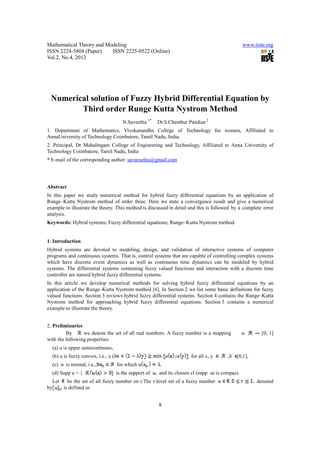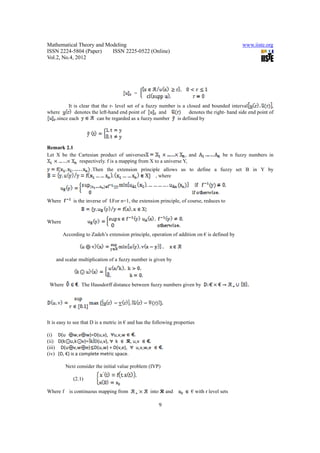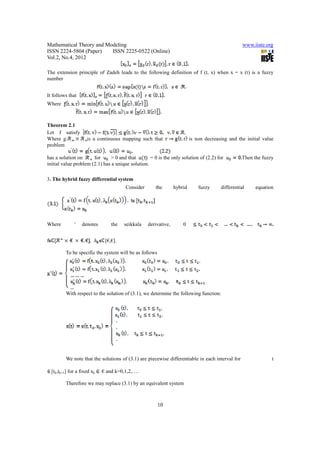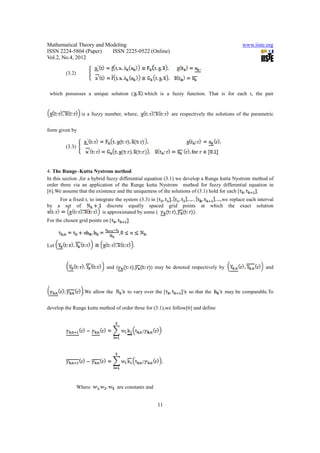Anzeige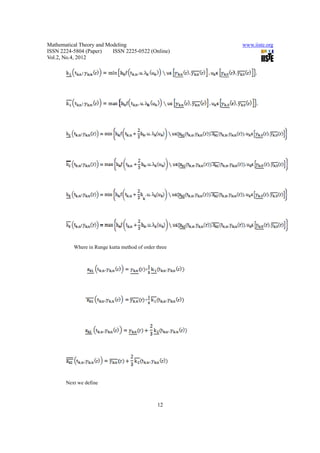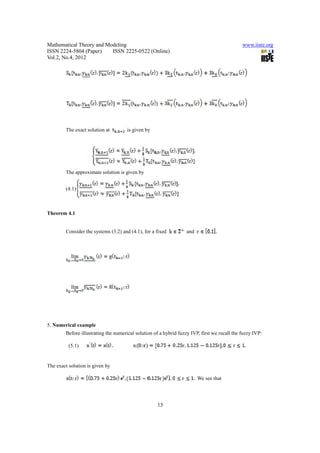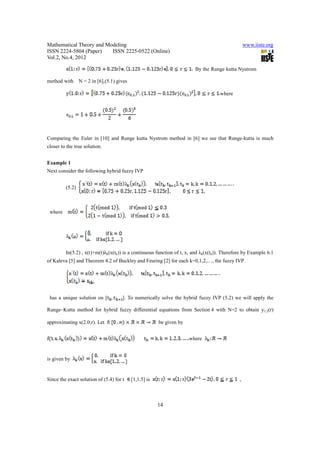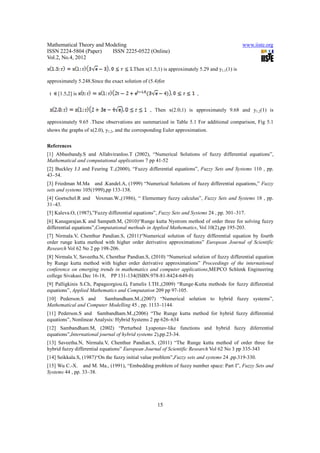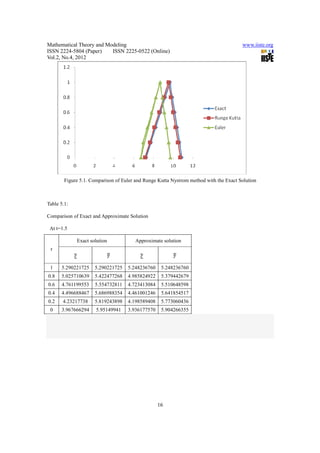Anzeige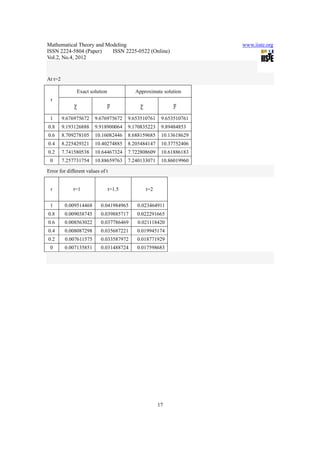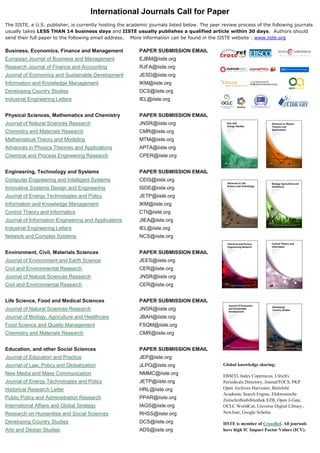Nächste SlideShare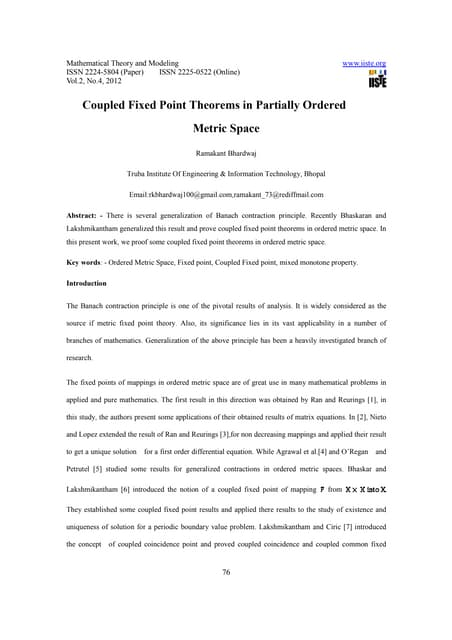11.coupled fixed point theorems in partially ordered metric space
1 von 11
Anzeige

### 11.numerical solution of fuzzy hybrid differential equation by third order runge kutta nystrom method

1. Mathematical Theory and Modeling www.iiste.org ISSN 2224-5804 (Paper) ISSN 2225-0522 (Online) Vol.2, No.4, 2012 Numerical solution of Fuzzy Hybrid Differential Equation by Third order Runge Kutta Nystrom Method N.Saveetha 1* Dr.S.Chenthur Pandian 2 1. Department of Mathematics, Vivekanandha College of Technology for women, Affiliated to AnnaUniversity of Technology Coimbatore, Tamil Nadu, India. 2. Principal, Dr Mahalingam College of Engineering and Technology, Affiliated to Anna University of Technology Coimbatore, Tamil Nadu, India * E-mail of the corresponding author: saveesethu@gmail.com Abstract In this paper we study numerical method for hybrid fuzzy differential equations by an application of Runge–Kutta Nystrom method of order three. Here we state a convergence result and give a numerical example to illustrate the theory. This method is discussed in detail and this is followed by a complete error analysis. Keywords: Hybrid systems; Fuzzy differential equations; Runge–Kutta Nystrom method 1. Introduction Hybrid systems are devoted to modeling, design, and validation of interactive systems of computer programs and continuous systems. That is, control systems that are capable of controlling complex systems which have discrete event dynamics as well as continuous time dynamics can be modeled by hybrid systems. The differential systems containing fuzzy valued functions and interaction with a discrete time controller are named hybrid fuzzy differential systems. In this article we develop numerical methods for solving hybrid fuzzy differential equations by an application of the Runge–Kutta Nystrom method . In Section 2 we list some basic definitions for fuzzy valued functions. Section 3 reviews hybrid fuzzy differential systems. Section 4 contains the Runge–Kutta Nystrom method for approaching hybrid fuzzy differential equations. Section 5 contains a numerical example to illustrate the theory. 2. Preliminaries By we denote the set of all real numbers. A fuzzy number is a mapping u: [0, 1] with the following properties: (a) u is upper semicontinous, (b) u is fuzzy convex, i.e., u ( for all x, y , [0,1], (c) is normal, i.e., for which (d) Supp u = { is the support of and its closure cl (supp ) is compact. Let be the set of all fuzzy number on r.The r-level set of a fuzzy number denoted by , is defined as 8
2. Mathematical Theory and Modeling www.iiste.org ISSN 2224-5804 (Paper) ISSN 2225-0522 (Online) Vol.2, No.4, 2012 = It is clear that the r- level set of a fuzzy number is a closed and bounded interval , where denotes the left-hand end point of and denotes the right- hand side end point of .since each can be regarded as a fuzzy number is defined by Remark 2.1 Let X be the Cartesian product of universes , and be n fuzzy numbers in respectively. f is a mapping from X to a universe Y, .Then the extension principle allows us to define a fuzzy set B in Y by , where Where is the inverse of .For n=1, the extension principle, of course, reduces to } Where According to Zadeh’s extension principle, operation of addition on € is defined by and scalar multiplication of a fuzzy number is given by Where The Hausdorff distance between fuzzy numbers given by It is easy to see that D is a metric in € and has the following properties (i) D(u w,v w)=D(u,v), u,v,w (ii) D(k u,k v)= D(u,v), k , u,v (iii) D(u v,w e) D(u,w) + D(v,e), u,v,w,e (iv) (D, €) is a complete metric space. Next consider the initial value problem (IVP) (2.1) Where f is continuous mapping from into and € with r level sets 9
3. Mathematical Theory and Modeling www.iiste.org ISSN 2224-5804 (Paper) ISSN 2225-0522 (Online) Vol.2, No.4, 2012 The extension principle of Zadeh leads to the following definition of f (t, x) when x = x (t) is a fuzzy number It follows that Where Theorem 2.1 Let f satisfy Where g: is a continuous mapping such that is non decreasing and the initial value problem has a solution on for > 0 and that = 0 is the only solution of (2.2) for Then the fuzzy initial value problem (2.1) has a unique solution. 3. The hybrid fuzzy differential system Consider the hybrid fuzzy differential equation Where denotes the seikkala derivative, 0 , [€,€]. To be specific the system will be as follows With respect to the solution of (3.1), we determine the following function: We note that the solutions of (3.1) are piecewise differentiable in each interval for t [tk,tk+1] for a fixed xk € and k=0,1,2,…. Therefore we may replace (3.1) by an equivalent system 10
4. Mathematical Theory and Modeling www.iiste.org ISSN 2224-5804 (Paper) ISSN 2225-0522 (Online) Vol.2, No.4, 2012 (3.2) which possesses a unique solution ( which is a fuzzy function. That is for each t, the pair is a fuzzy number, where, are respectively the solutions of the parametric form given by (3.3) 4. The Runge–Kutta Nystrom method In this section ,for a hybrid fuzzy differential equation (3.1) we develop a Runge kutta Nystrom method of order three via an application of the Runge kutta Nystrom method for fuzzy differential equation in .We assume that the existence and the uniqueness of the solutions of (3.1) hold for each [ . For a fixed r, to integrate the system (3.3) in [ we replace each interval by a set of discrete equally spaced grid points at which the exact solution is approximated by some ( , . For the chosen grid points on [ , , Let . and ( , ) may be denoted respectively by and .We allow the to vary over the [ so that the may be comparable.To develop the Runge kutta method of order three for (3.1),we follow and define Where are constants and 11
5. Mathematical Theory and Modeling www.iiste.org ISSN 2224-5804 (Paper) ISSN 2225-0522 (Online) Vol.2, No.4, 2012 Where in Runge kutta method of order three + + Next we define 12
6. Mathematical Theory and Modeling www.iiste.org ISSN 2224-5804 (Paper) ISSN 2225-0522 (Online) Vol.2, No.4, 2012 The exact solution at is given by The approximate solution is given by (4.1) Theorem 4.1 Consider the systems (3.2) and (4.1), for a fixed and 5. Numerical example Before illustrating the numerical solution of a hybrid fuzzy IVP, first we recall the fuzzy IVP: (5.1) The exact solution is given by We see that 13
7. Mathematical Theory and Modeling www.iiste.org ISSN 2224-5804 (Paper) ISSN 2225-0522 (Online) Vol.2, No.4, 2012 By the Runge kutta Nystrom method with N = 2 in ,(5.1) gives where Comparing the Euler in  and Runge kutta Nystrom method in  we see that Runge-kutta is much closer to the true solution. Example 1 Next consider the following hybrid fuzzy IVP (5.2) where In(5.2) , x(t)+m(t)λk(x(tk)) is a continuous function of t, x, and λk(x(tk)). Therefore by Example 6.1 of Kaleva  and Theorem 4.2 of Buckley and Feuring  for each k=0,1,2,…, the fuzzy IVP has a unique solution on [ ]. To numerically solve the hybrid fuzzy IVP (5.2) we will apply the Runge–Kutta method for hybrid fuzzy differential equations from Section 4 with N=2 to obtain y1,2(r) approximating x(2.0;r). Let be given by where is given by Since the exact solution of (5.4) for t [1,1.5] is , 14
8. Mathematical Theory and Modeling www.iiste.org ISSN 2224-5804 (Paper) ISSN 2225-0522 (Online) Vol.2, No.4, 2012 Then x(1.5;1) is approximately 5.29 and y1,1(1) is approximately 5.248.Since the exact solution of (5.4)for t [1.5,2] is , , Then x(2.0;1) is approximately 9.68 and y1,2(1) is approximately 9.65 .These observations are summarized in Table 5.1 For additional comparison, Fig 5.1 shows the graphs of x(2.0), y1,2, and the corresponding Euler approximation. References  Abbasbandy.S and Allahviranloo.T (2002), “Numerical Solutions of fuzzy differential equations”, Mathematical and computational applications 7 pp 41-52  Buckley J.J and Feuring T.,(2000), “Fuzzy differential equations”, Fuzzy Sets and Systems 110 , pp. 43–54.  Friedman M.Ma and .Kandel.A, (1999) “Numerical Solutions of fuzzy differential equations,” Fuzzy sets and systems 105(1999),pp 133-138.  Goetschel.R and Voxman.W.,(1986), “ Elementary fuzzy calculus”, Fuzzy Sets and Systems 18 , pp. 31–43.  Kaleva.O, (1987),”Fuzzy differential equations”, Fuzzy Sets and Systems 24 , pp. 301–317.  Kanagarajan.K and Sampath.M, (2010)“Runge kutta Nystrom method of order three for solving fuzzy differential equations”,Computational methods in Applied Mathematics, Vol 10(2),pp 195-203.  Nirmala.V, Chenthur Pandian.S, (2011)“Numerical solution of fuzzy differential equation by fourth order runge kutta method with higher order derivative approximations” European Journal of Scientific Research Vol 62 No 2 pp 198-206.  Nirmala.V, Saveetha.N, Chenthur Pandian.S, (2010) “Numerical solution of fuzzy differential equation by Runge kutta method with higher order derivative approximations” Proceedings of the international conference on emerging trends in mathematics and computer applications,MEPCO Schlenk Engineering college Sivakasi.Dec 16-18, PP 131-134(ISBN:978-81-8424-649-0)  Palligkinis S.Ch, Papageorgiou.G, Famelis I.TH.,(2009) “Runge-Kutta methods for fuzzy differential equations”, Applied Mathematics and Computation 209 pp 97-105.  Pederson.S and Sambandham.M.,(2007) “Numerical solution to hybrid fuzzy systems”, Mathematical and Computer Modelling 45 , pp. 1133–1144.  Pederson.S and Sambandham.M.,(2006) “The Runge kutta method for hybrid fuzzy differential equations”, Nonlinear Analysis: Hybrid Systems 2 pp 626–634  Sambandham.M, (2002) “Perturbed Lyaponav-like functions and hybrid fuzzy diferrential equations”,International journal of hybrid systems 2),pp.23-34.  Saveetha.N, Nirmala.V, Chenthur Pandian.S, (2011) “The Runge kutta method of order three for hybrid fuzzy differential equations” European Journal of Scientific Research Vol 62 No 3 pp 335-343  Seikkala.S, (1987)“On the fuzzy initial value problem”,Fuzzy sets and systems 24 ,pp.319-330.  Wu C.-X. and M. Ma., (1991), “Embedding problem of fuzzy number space: Part I”, Fuzzy Sets and Systems 44 , pp. 33–38. 15
9. Mathematical Theory and Modeling www.iiste.org ISSN 2224-5804 (Paper) ISSN 2225-0522 (Online) Vol.2, No.4, 2012 Figure 5.1. Comparison of Euler and Runge Kutta Nystrom method with the Exact Solution Table 5.1: Comparison of Exact and Approximate Solution At t=1.5 Exact solution Approximate solution r 1 5.290221725 5.290221725 5.248236760 5.248236760 0.8 5.025710639 5.422477268 4.985824922 5.379442679 0.6 4.761199553 5.554732811 4.723413084 5.510648598 0.4 4.496688467 5.686988354 4.461001246 5.641854517 0.2 4.23217738 5.819243898 4.198589408 5.773060436 0 3.967666294 5.95149941 3.936177570 5.904266355 16
10. Mathematical Theory and Modeling www.iiste.org ISSN 2224-5804 (Paper) ISSN 2225-0522 (Online) Vol.2, No.4, 2012 At t=2 Exact solution Approximate solution r 1 9.676975672 9.676975672 9.653510761 9.653510761 0.8 9.193126888 9.918900064 9.170835223 9.89484853 0.6 8.709278105 10.16082446 8.688159685 10.13618629 0.4 8.225429321 10.40274885 8.205484147 10.37752406 0.2 7.741580538 10.64467324 7.722808609 10.61886183 0 7.257731754 10.88659763 7.240133071 10.86019960 Error for different values of t r t=1 t=1.5 t=2 1 0.009514468 0.041984965 0.023464911 0.8 0.009038745 0.039885717 0.022291665 0.6 0.008563022 0.037786469 0.021118420 0.4 0.008087298 0.035687221 0.019945174 0.2 0.007611575 0.033587972 0.018771929 0 0.007135851 0.031488724 0.017598683 17